Скачать презентацию We re Going to the Store By Maranda White

a241251707af46442887563be5566dd5.ppt

• Количество слайдов: 30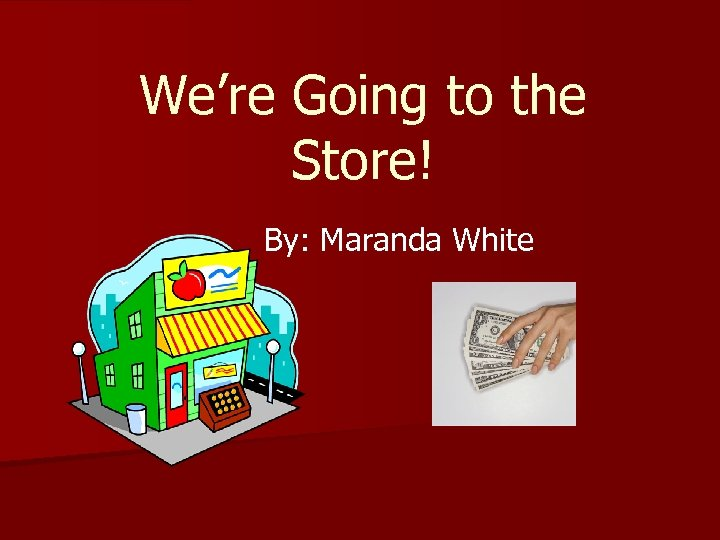We’re Going to the Store! By: Maranda White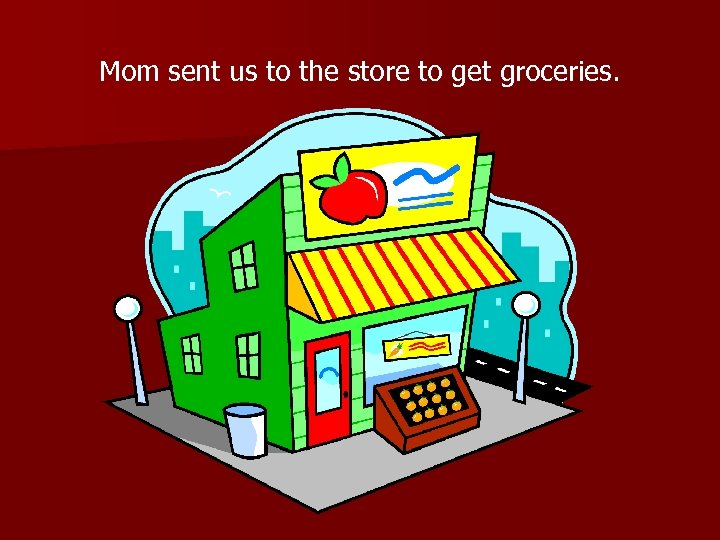Mom sent us to the store to get groceries.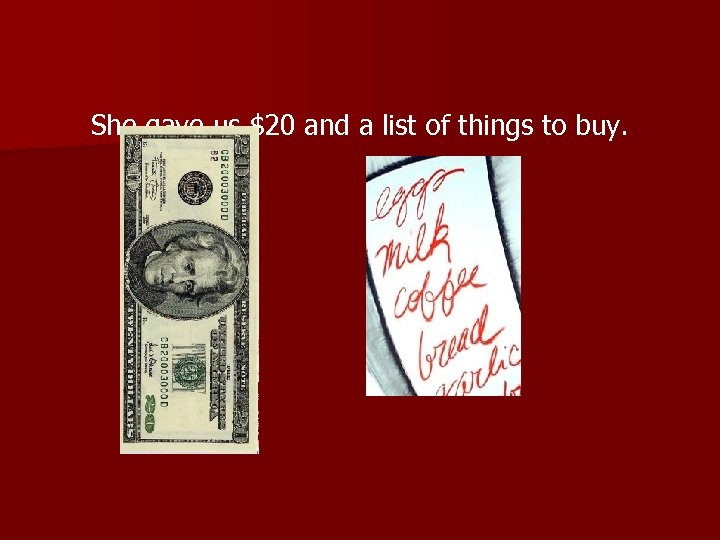She gave us \$20 and a list of things to buy.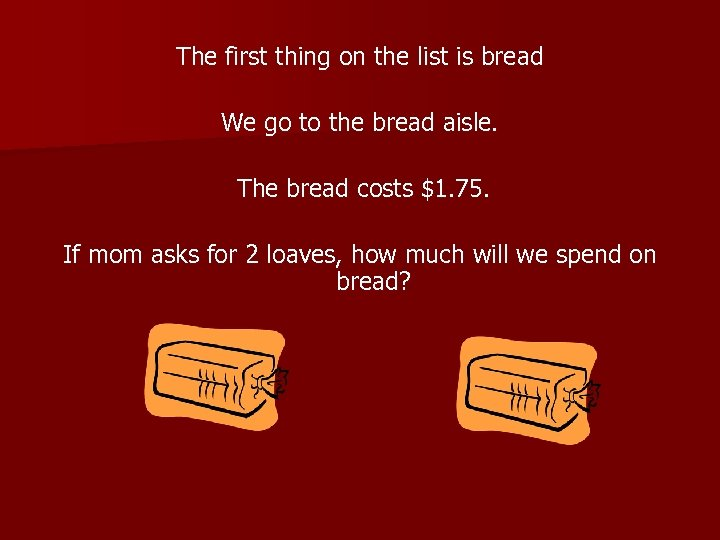The first thing on the list is bread We go to the bread aisle. The bread costs \$1. 75. If mom asks for 2 loaves, how much will we spend on bread?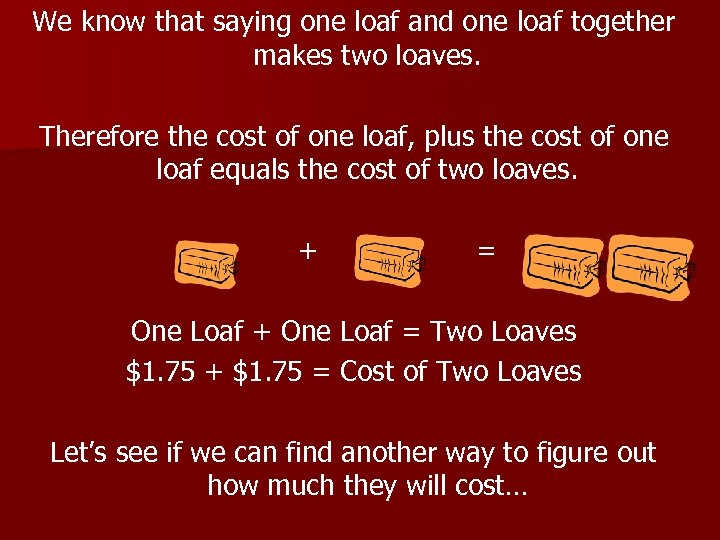We know that saying one loaf and one loaf together makes two loaves. Therefore the cost of one loaf, plus the cost of one loaf equals the cost of two loaves. + = One Loaf + One Loaf = Two Loaves \$1. 75 + \$1. 75 = Cost of Two Loaves Let’s see if we can find another way to figure out how much they will cost…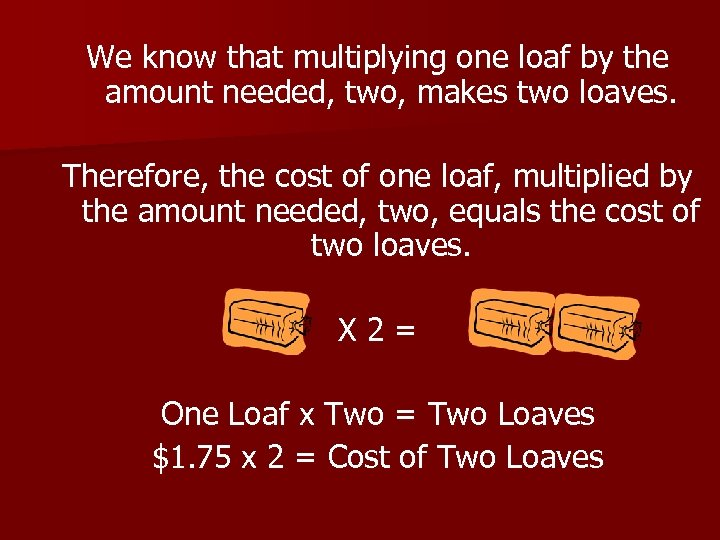We know that multiplying one loaf by the amount needed, two, makes two loaves. Therefore, the cost of one loaf, multiplied by the amount needed, two, equals the cost of two loaves. X 2= One Loaf x Two = Two Loaves \$1. 75 x 2 = Cost of Two Loaves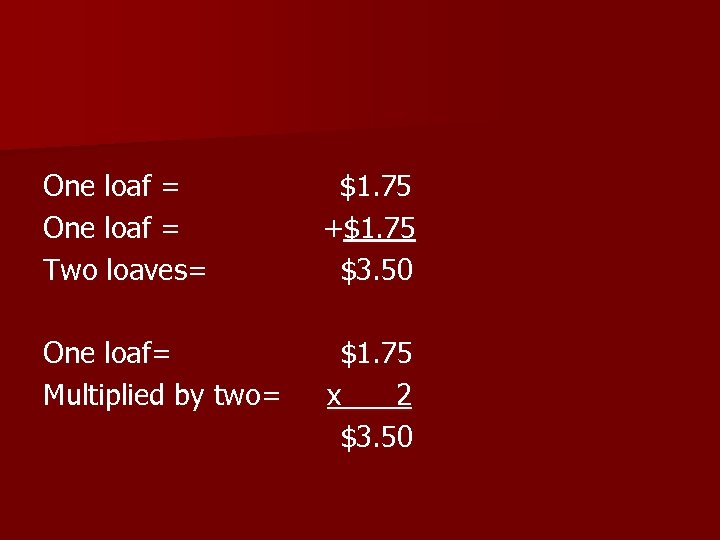One loaf = Two loaves= \$1. 75 +\$1. 75 \$3. 50 One loaf= Multiplied by two= \$1. 75 x 2 \$3. 50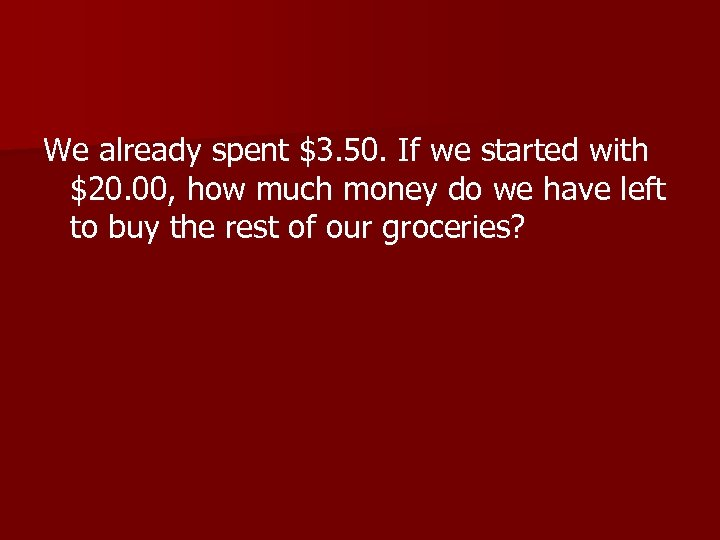We already spent \$3. 50. If we started with \$20. 00, how much money do we have left to buy the rest of our groceries?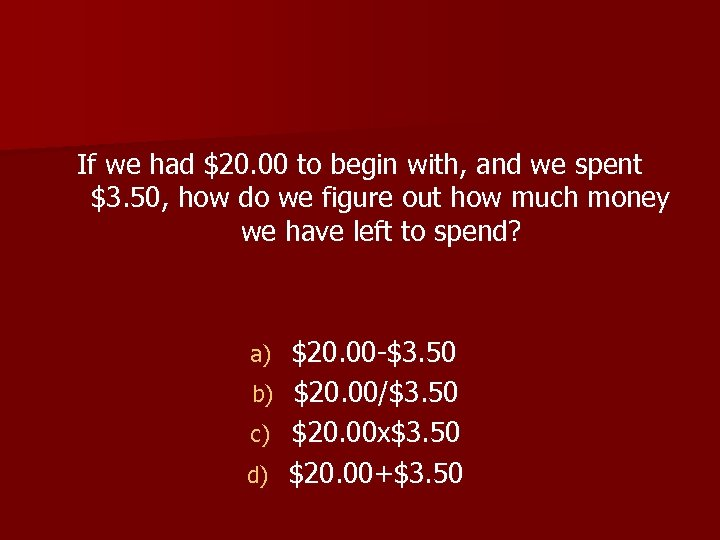If we had \$20. 00 to begin with, and we spent \$3. 50, how do we figure out how much money we have left to spend? \$20. 00 -\$3. 50 b) \$20. 00/\$3. 50 c) \$20. 00 x\$3. 50 d) \$20. 00+\$3. 50 a)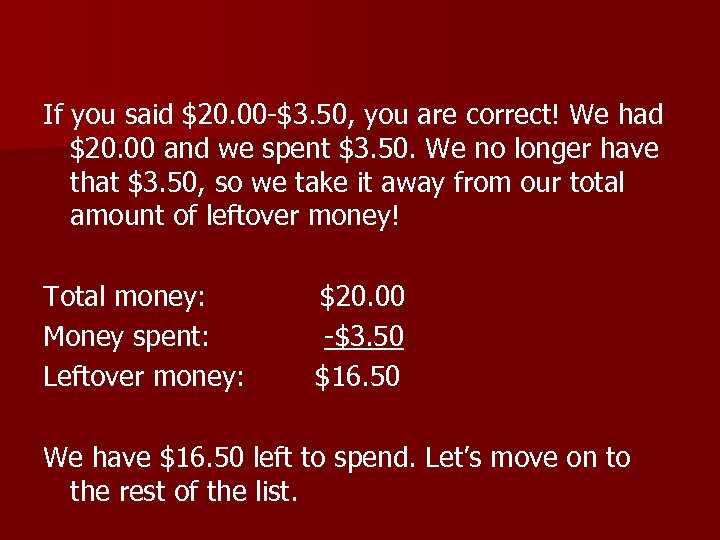If you said \$20. 00 -\$3. 50, you are correct! We had \$20. 00 and we spent \$3. 50. We no longer have that \$3. 50, so we take it away from our total amount of leftover money! Total money: Money spent: Leftover money: \$20. 00 -\$3. 50 \$16. 50 We have \$16. 50 left to spend. Let’s move on to the rest of the list.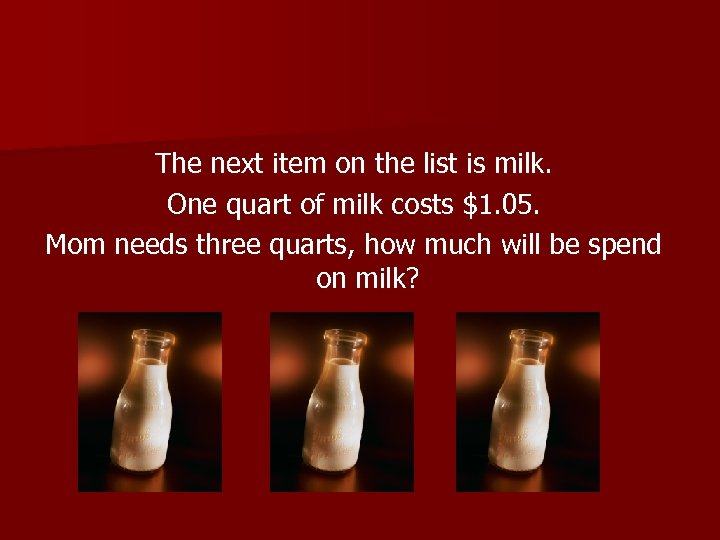The next item on the list is milk. One quart of milk costs \$1. 05. Mom needs three quarts, how much will be spend on milk?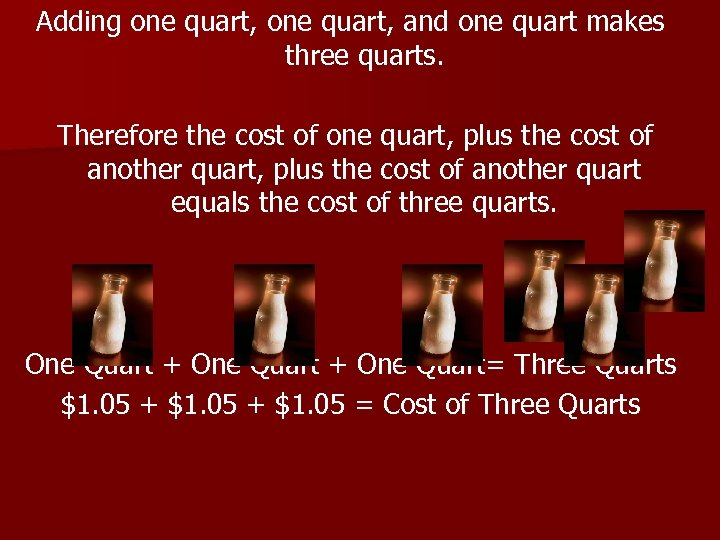Adding one quart, and one quart makes three quarts. Therefore the cost of one quart, plus the cost of another quart equals the cost of three quarts. One Quart + One Quart= Three Quarts \$1. 05 + \$1. 05 = Cost of Three Quarts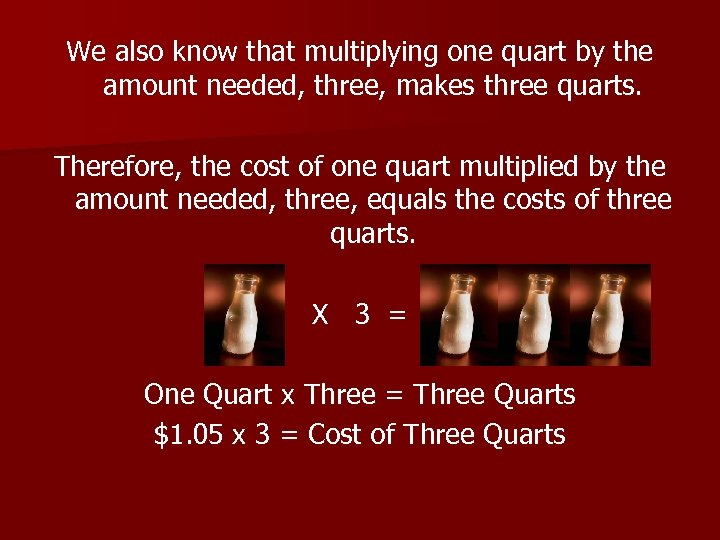We also know that multiplying one quart by the amount needed, three, makes three quarts. Therefore, the cost of one quart multiplied by the amount needed, three, equals the costs of three quarts. X 3 = One Quart x Three = Three Quarts \$1. 05 x 3 = Cost of Three Quarts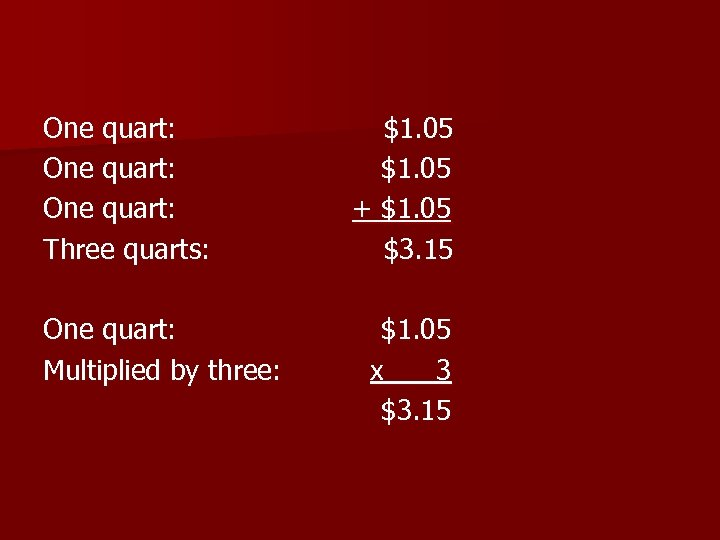One quart: Three quarts: One quart: Multiplied by three: \$1. 05 + \$1. 05 \$3. 15 \$1. 05 x 3 \$3. 15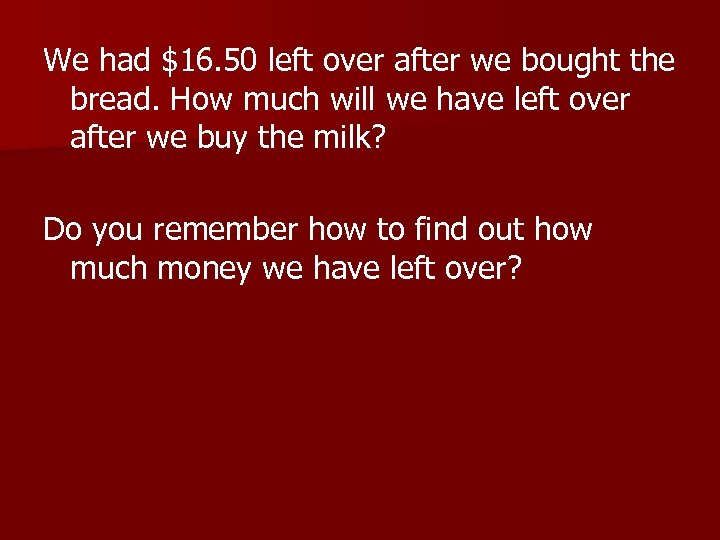We had \$16. 50 left over after we bought the bread. How much will we have left over after we buy the milk? Do you remember how to find out how much money we have left over?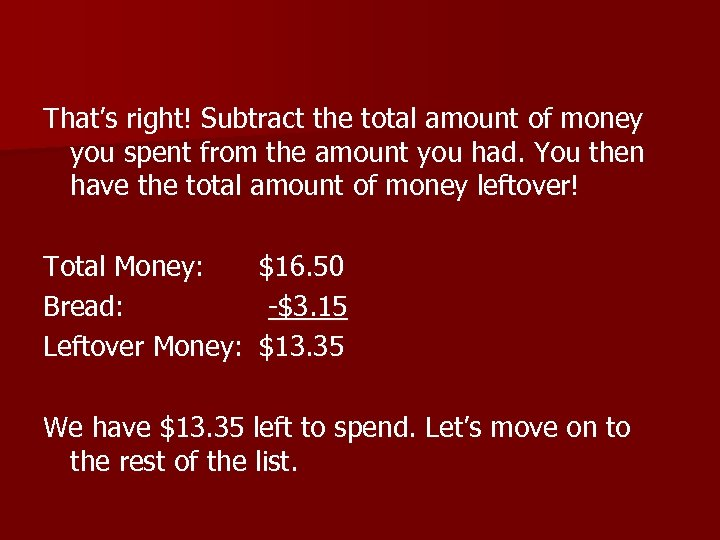That’s right! Subtract the total amount of money you spent from the amount you had. You then have the total amount of money leftover! Total Money: Bread: Leftover Money: \$16. 50 -\$3. 15 \$13. 35 We have \$13. 35 left to spend. Let’s move on to the rest of the list.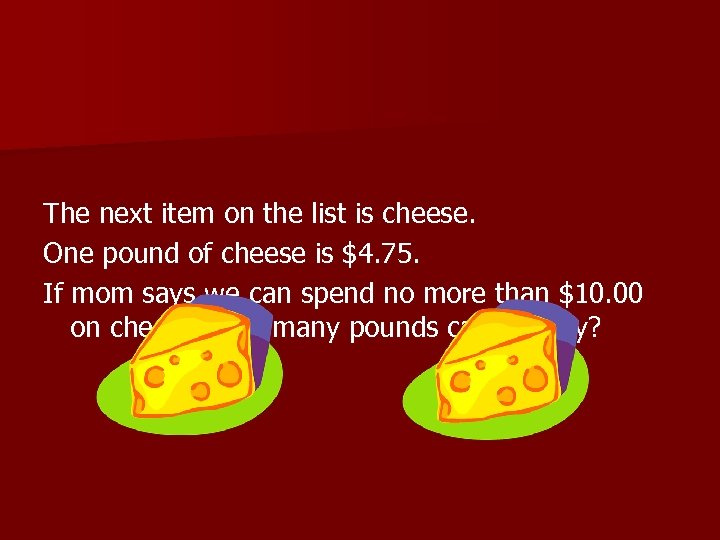The next item on the list is cheese. One pound of cheese is \$4. 75. If mom says we can spend no more than \$10. 00 on cheese, how many pounds can we buy?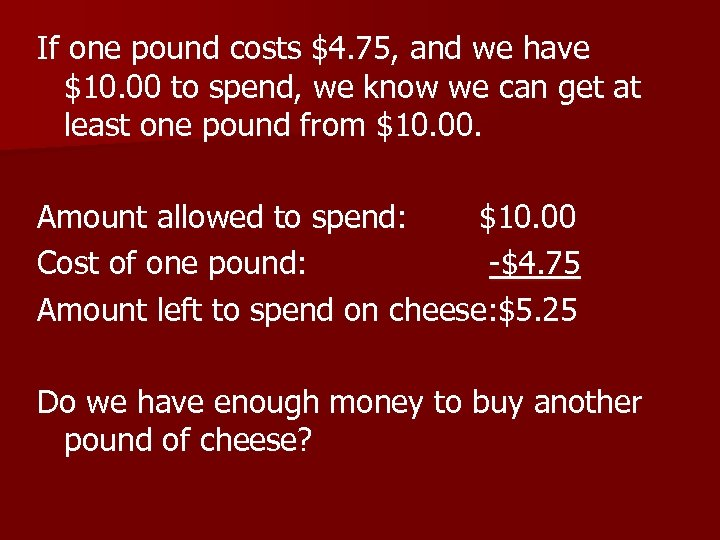If one pound costs \$4. 75, and we have \$10. 00 to spend, we know we can get at least one pound from \$10. 00. Amount allowed to spend: \$10. 00 Cost of one pound: -\$4. 75 Amount left to spend on cheese: \$5. 25 Do we have enough money to buy another pound of cheese?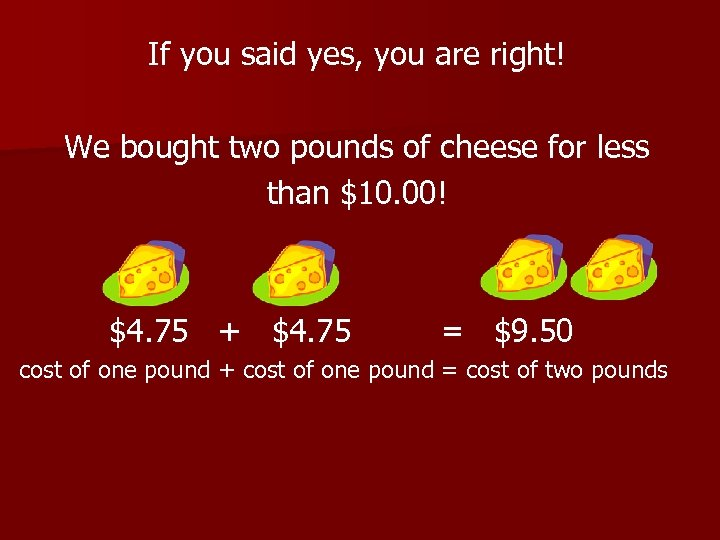If you said yes, you are right! We bought two pounds of cheese for less than \$10. 00! \$4. 75 + \$4. 75 = \$9. 50 cost of one pound + cost of one pound = cost of two pounds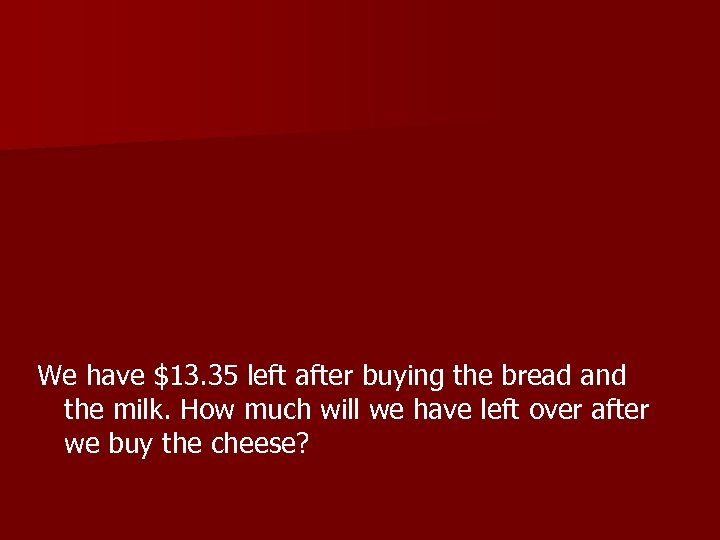We have \$13. 35 left after buying the bread and the milk. How much will we have left over after we buy the cheese?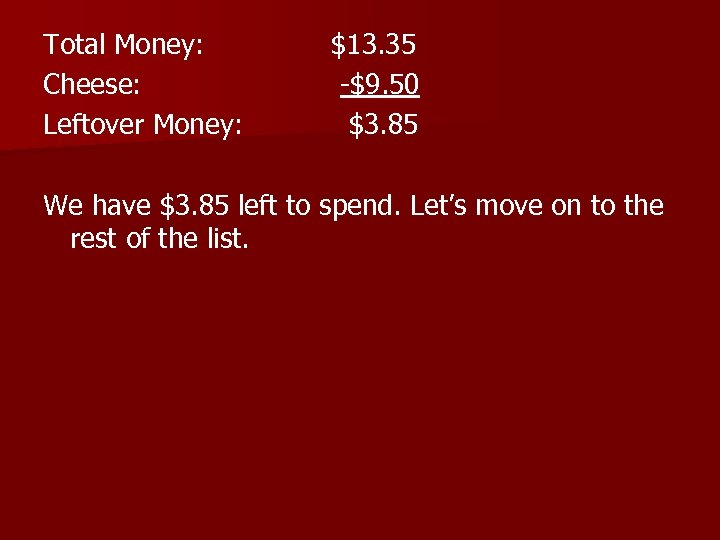Total Money: Cheese: Leftover Money: \$13. 35 -\$9. 50 \$3. 85 We have \$3. 85 left to spend. Let’s move on to the rest of the list.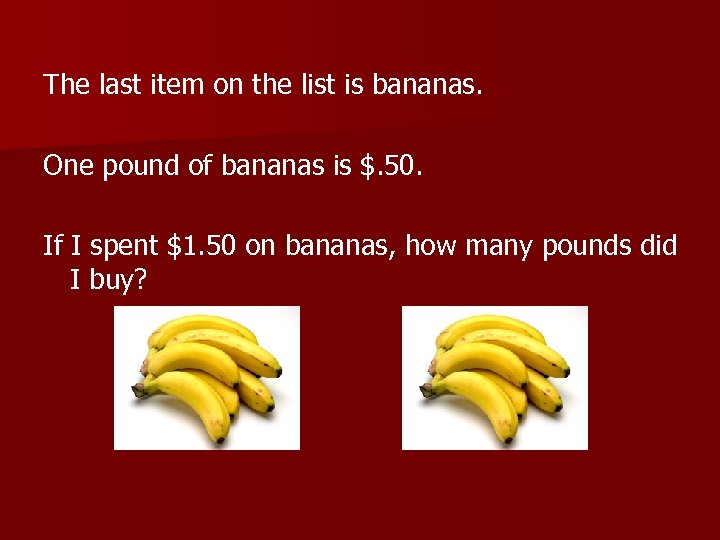The last item on the list is bananas. One pound of bananas is \$. 50. If I spent \$1. 50 on bananas, how many pounds did I buy?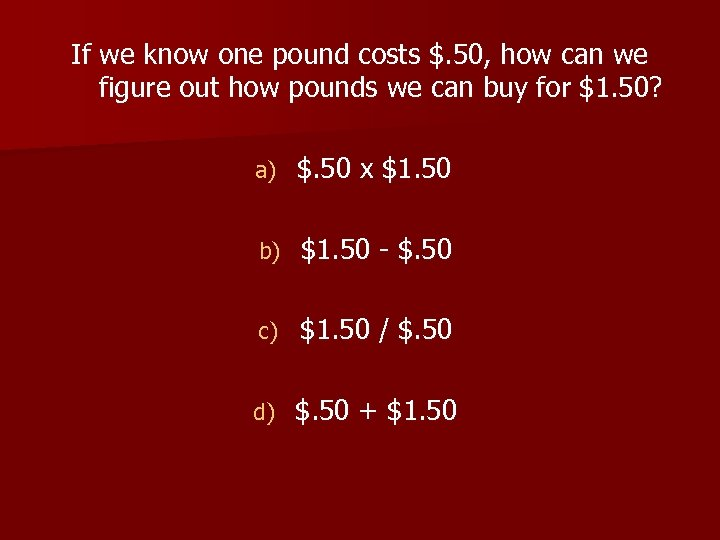If we know one pound costs \$. 50, how can we figure out how pounds we can buy for \$1. 50? a) \$. 50 x \$1. 50 b) \$1. 50 - \$. 50 c) \$1. 50 / \$. 50 d) \$. 50 + \$1. 50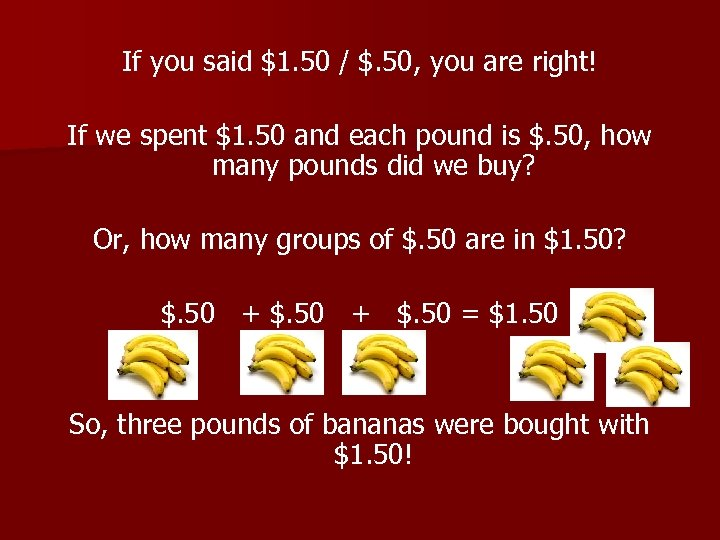If you said \$1. 50 / \$. 50, you are right! If we spent \$1. 50 and each pound is \$. 50, how many pounds did we buy? Or, how many groups of \$. 50 are in \$1. 50? \$. 50 + \$. 50 = \$1. 50 So, three pounds of bananas were bought with \$1. 50!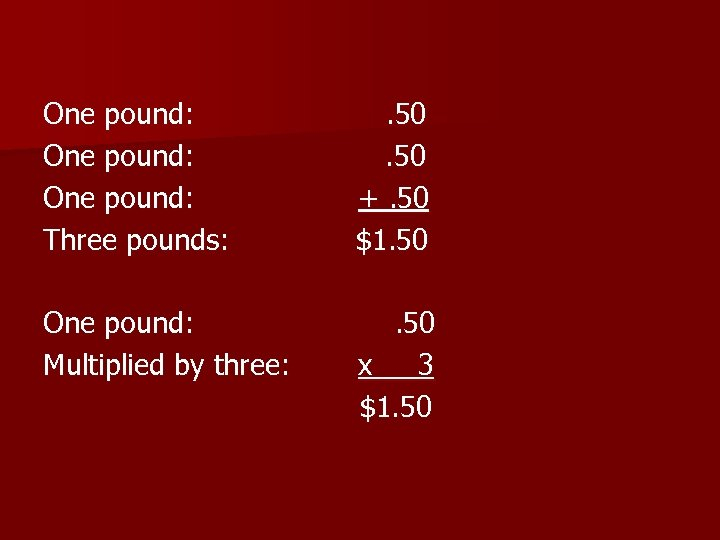One pound: Three pounds: . 50 +. 50 \$1. 50 One pound: Multiplied by three: . 50 x 3 \$1. 50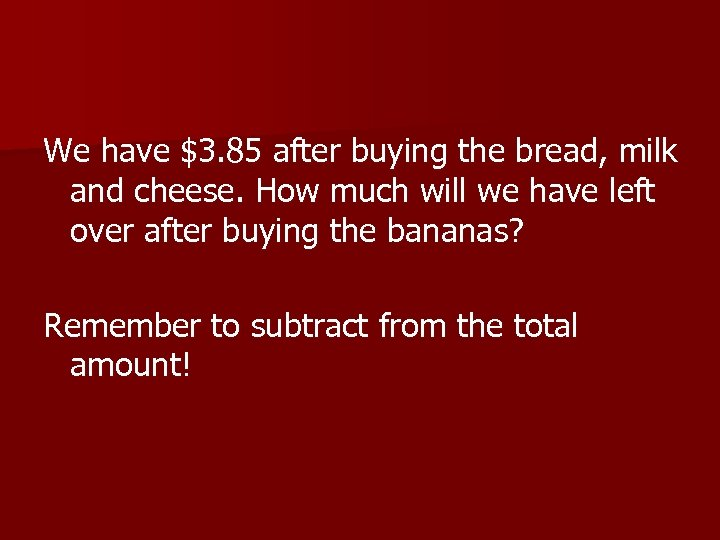We have \$3. 85 after buying the bread, milk and cheese. How much will we have left over after buying the bananas? Remember to subtract from the total amount!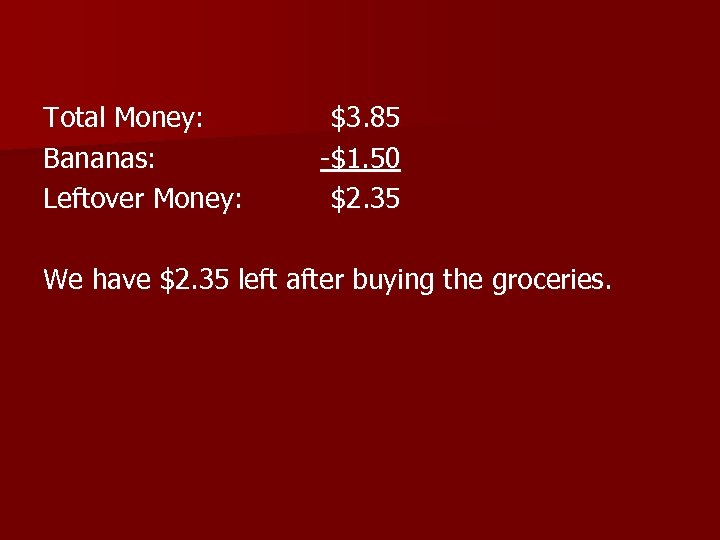Total Money: Bananas: Leftover Money: \$3. 85 -\$1. 50 \$2. 35 We have \$2. 35 left after buying the groceries.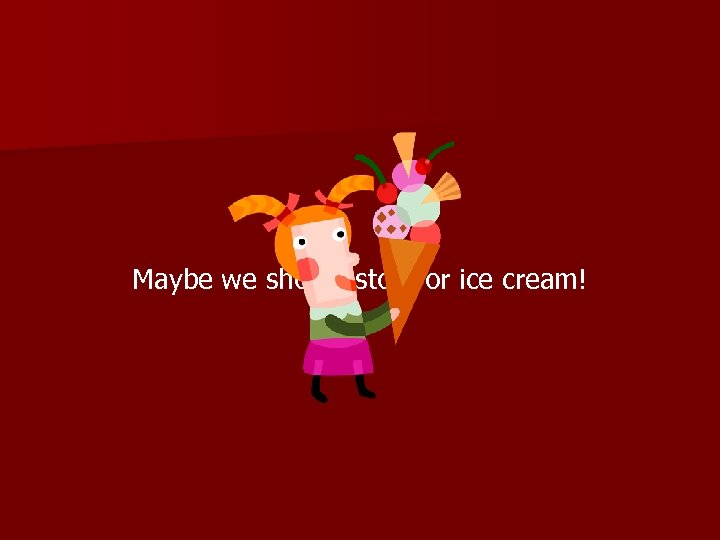Maybe we should stop for ice cream!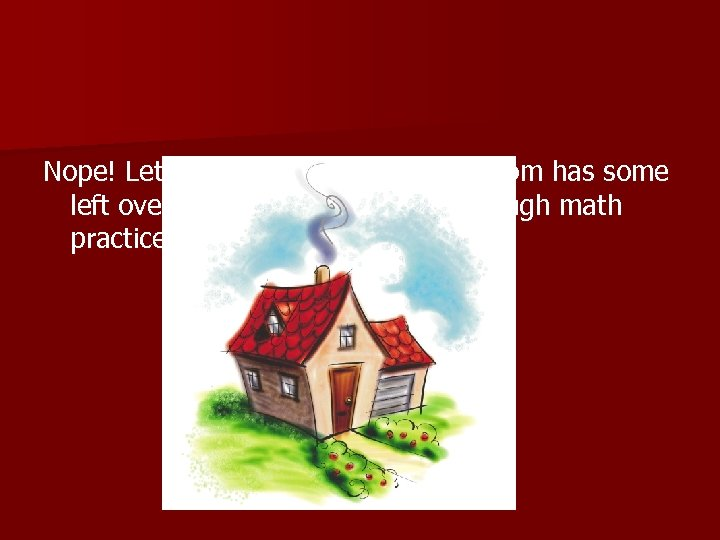Nope! Let’s just go home! I’m sure mom has some left over in the fridge- I’ve had enough math practice for today!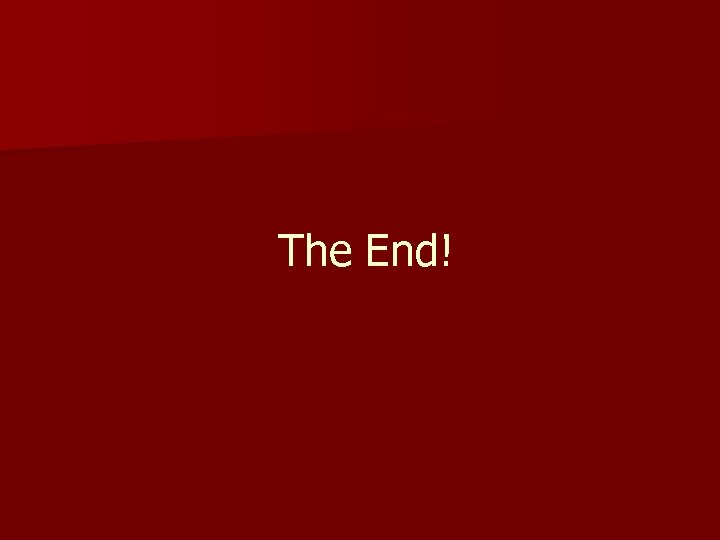The End!